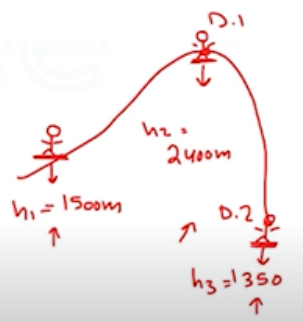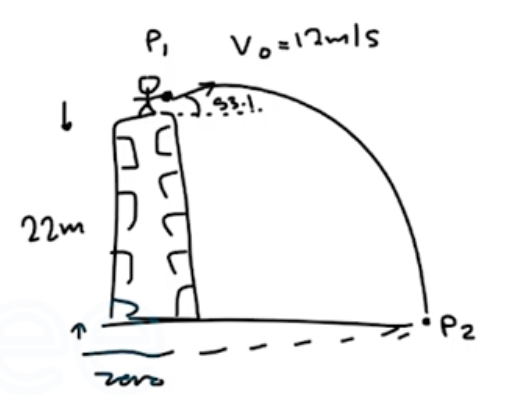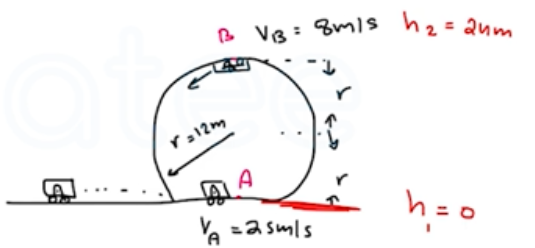Need Help?

Subscribe to Physics 1

###### \${selected_topic_name}
• Notes

$\begin{array}{l}{\text { In one day, a } 75-\mathrm{kg} \text { mountain climber ascends from the }} \\ {1500 \text { -m level on a vertical cliff to the top at } 2400 \mathrm{m} \text { . The next day, }} \\ {\text { she descends from the top to the base of the cliff, which is at an }} \\ {\text { elevation of } 1350 \mathrm{m} \text { . What is her change in gravitational potential }} \\ {\text { energy (a) on the first day and (b) on the second day? }}\end{array}$

$U=m g h$

$U_{\text {begin }}=m g h_{1} \quad, \quad U_{\text {day1 }}=m g h_{2}\quad, \quad U_{\text {day2 }}={mgh}_{3}$

$\Delta U_{da y 1} ? ?$$\Delta U _{day1}=m g h_{2}-m g h_{1}$

$=m g\left(h_{2}-h_{1}\right)=(75)(9.8)(2400-1500)$

$\Delta U_{day1}=6.6 * 10^{5} J$

$\Delta U_\text { day2 } ??$

$\Delta U_\text { day2 }=m g h_{3}-m g h_{2}=m g\left(h_{3}-h_{1}\right)=75(9.8)(1350-2400)$

$\Delta U_{da y_{2}}=-7.7*10^{5} \mathrm{J}$

$\begin{array}{l}{\text { A baseball is thrown from the roof of a } 22.0 \text { -m-tall building }} \\ {\text { with an initial velocity of magnitude } 12.0 \mathrm{m} / \mathrm{s} \text { and directed at an }} \\ {\text { angle of } 53.1^{\circ} \text { above the horizontal. (a) What is the speed of the }} \\ {\text { ball just before it strikes the ground? Use energy methods and }} \\ {\text { ignore air resistance. (b) What is the answer for part (a) if the in- }} \\ {\text { tial velocity is at an angle of } 53.1^{\circ} \text { below the horizontal? (c) If the }} \\ {\text { effects of air resistance are included, will part (a) or (b) give the }} \\ {\text { higher speed? }}\end{array}$(a) $\left[K_{1}+U_{1}+W_{\text {others }}\right]=k_{2}+U_{2}$

$K_{1}=\frac{1}{2} m {v_{1}}^{2} \rightarrow V_{1}=12 m / s$

$k_{2}=\frac{1}{2} m {v_{2}}^{2} \rightarrow v_{2}=? ?$

$U_{1}=m g h_{1} \quad \longrightarrow h_{1}=22 m$

$U_{2}=m g h_{2} \rightarrow h_{2}=0$

$W_\text { others }=0 \quad \longrightarrow \text { air res. }$

$\rightarrow \frac{1}{2} m(12)^{2}+m(9.8)(22)+0=\frac{1}{2} m{ v_{2}}^{2}+0$

$\rightarrow \frac{1}{2} m(12)^{2}+m(9.8)(22)=\frac{1}{2} m {v_{2}}^{2}\longrightarrow \frac{1}{2}(12)^{2}+(9.8)(22)=\frac{1}{2}{ V_{2}}^{2}$

$∴ 287.6=\frac{1}{2}{ v_{2}}^{2} \rightarrow V_{2}=\sqrt{575.2}=24 \mathrm{m/s}$

$V_{2} \longrightarrow 24 \mathrm{m} / \mathrm{s}$

$\begin{array}{l}{\text { You are testing a new amusement park roller coaster with }} \\ {\text { an empty car of mass } 120 \mathrm{kg} \text { . One part of the track is a vertical }} \\ {\text { loop with radius } 12.0 \mathrm{m} \text { . At the bottom of the loop (point } A) \text { the car }} \\ {\text { has speed } 25.0 \mathrm{m} / \mathrm{s} \text { , and at the top of the loop (point } B \text { ) it has speed }} \\ {8.0 \mathrm{m} / \mathrm{s} \text { . As the car rolls from point } A \text { to point } B \text { , how much work }} \\ {\text { is done by friction? }}\end{array}$$K_{1}+U_{1}+W_{\text {others }}=K_{2}+U_{2}$

$k_{1}=\frac{1}{2} m {v_1}^{2}=\frac{1}{2}(120)(25)^{2}=37500J$

$U_{1}=0$

$K_{2}=\frac{1}{2} m {v_{2}}^{2}=\frac{1}{2}(120)(8)^{2}=3840 \mathrm{J}$

$U_{2}=m g h_{2}=120 \times 9.8 \times 24=28224 \mathrm{J}$

$\text { Wothers } ? ?$

$\rightarrow 37500+W_{others}=3840+28224$

$W_\text { others }=3840+28224-37500=-5400 J$